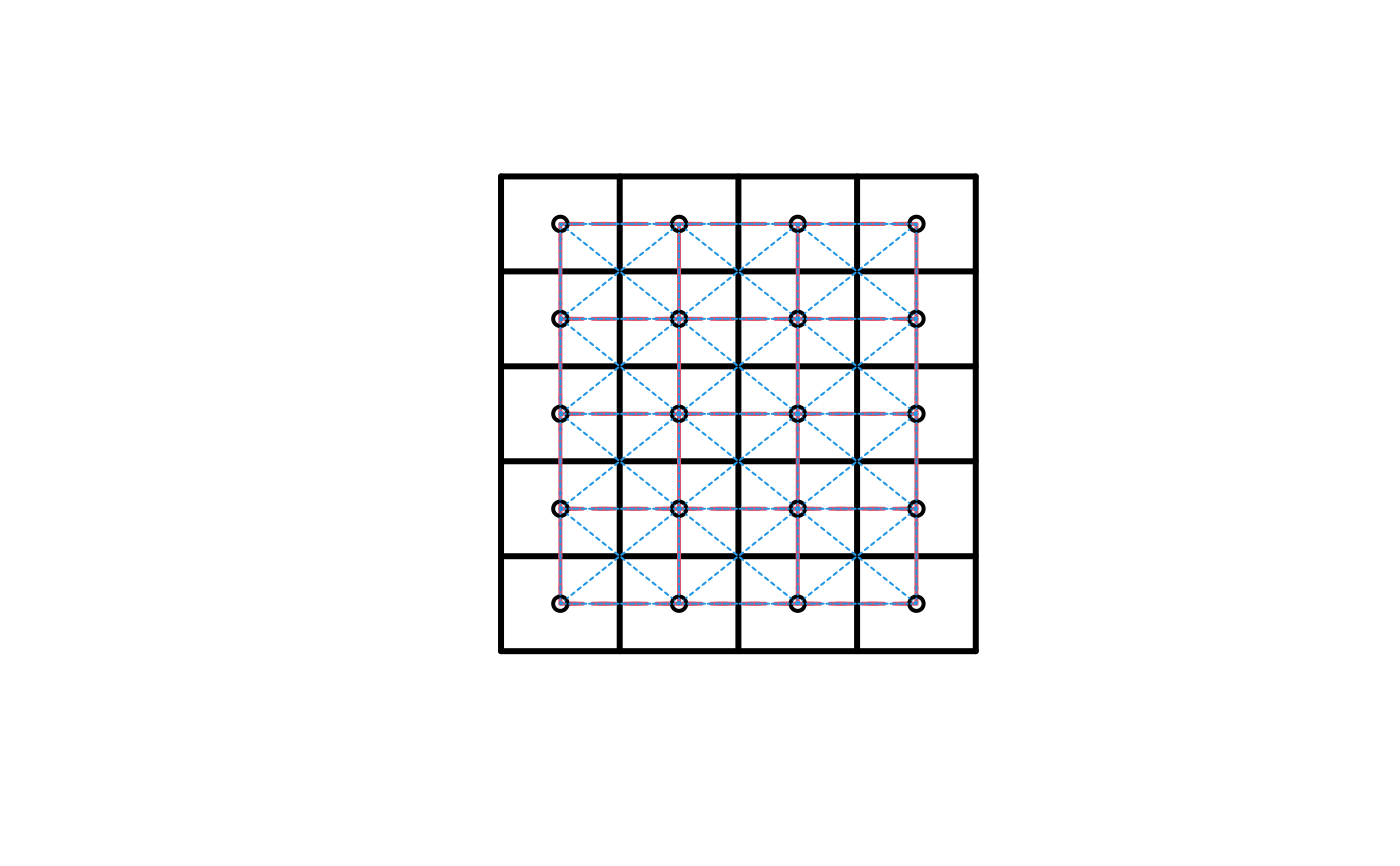The function builds a neighbours list for a grid topology. It works for a k-dimentional grid topology, k>=1.

grid2nb(grid, d = grid@cells.dim,
queen = TRUE, nb = TRUE, self = FALSE)

## Arguments

grid

An object of class GridTopology. One can avoid to supply this by just suplying the dimentions in the d argument.

d

A scalar (for one dimentional grid) or a length k vector specyfying the number of grid cells in each direction of the k dimentions.

queen

Logical. Default is TRUE. To inform if the queen neighbourhood structure should be considered. If FALSE, only a hyper-cube with a common face will be considered neighbour. If TRUE, a single shared coordinate meets the contiguity condition.

nb

Default TRUE. If TRUE, return the result as a neighbours list with class nb. If FALSE, the result is a matrix with 3^k columns if self = TRUE or 3^k-1 if self = FALSE. Zeros are used for hyper-cubes at boundaries.

self

Default FALSE, to indicate if the hyper-cube neighbour itself should be considered a neighbour.

## Value

Either a matrix, if “nb” is FALSE or a neighbours list with class nb. See card for details of “nb”

objects.

## Note

This applies to a k-dimentional grid topology.

## Author

poly2nb, summary.nb, card

## Examples

nb <- grid2nb(d = c(5L, 5L, 5L))
nb
#> Neighbour list object:
#> Number of regions: 125
#> Number of nonzero links: 2072
#> Percentage nonzero weights: 13.2608
#> Average number of links: 16.576
summary(nb)
#> Neighbour list object:
#> Number of regions: 125
#> Number of nonzero links: 2072
#> Percentage nonzero weights: 13.2608
#> Average number of links: 16.576
#>
#>  7 11 17 26
#>  8 36 54 27
#> 8 least connected regions:
#> 1 5 21 25 101 105 121 125 with 7 links
#> 27 most connected regions:
#> 32 33 34 37 38 39 42 43 44 57 58 59 62 63 64 67 68 69 82 83 84 87 88 89 92 93 94 with 26 links

gt <- GridTopology(c(.125,.1), c(.25,.2), c(4L, 5L))
nb1 <- grid2nb(gt, queen = FALSE)
nb2 <- grid2nb(gt)

sg <- SpatialGrid(gt)
plot(sg, lwd=3)
plot(nb1, coordinates(sg), add=TRUE, lty=2, col=2, lwd=2)#>  $: int 2 #>$ : int [1:2] 1 3
#>  $: int [1:2] 2 4 #>$ : int [1:2] 3 5